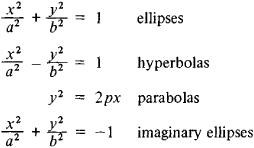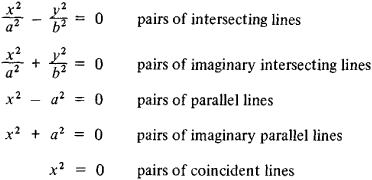a plane curve whose rectangular Cartesian coordinates satisfy a second-degree algebraic equation

(*) a11x2 + 2a12xy + a22y2 + 2a13x + 2a23y + a33 = 0

The equation (*) may not define a figure in the real plane. Then, to preserve generality, we say that (*) defines an imaginary quadratic curve. Depending on the values of the coefficients of the general equation (*), it can be transformed by a translation of the origin and a rotation of the coordinate system to one of the nine canonical forms given below, each of which determines a certain class of curves. Namely,

(1) irreducible curves:(2) reducible curves:The nature of quadratic curves can be determined without reducing the general equation to a canonical form. Instead we examine the values of the fundamental invariants of quadratic curves— expressions formed from the terms of equation (*) whose values are not affected by translations and rotations of the coordinate system. The invariants in question areThus, for example, as irreducible curves, ellipses are characterized by the fact that for them Δ ≠ 0; the positive value of the invariant δ differentiates between ellipses and other types of irreducible curves (for hyperbolas, δ < 0; for parabolas, δ = 0). Comparison of the signs of the invariants Δ and S makes it possible to distinguish between real and imaginary ellipses: if Δ and S have different signs, then the ellipse is real; it is imaginary if Δ and S have the same sign.

The three fundamental invariants Δ, δ, and S define quadratic curves (except for parallel lines) to within a motion of the Euclidean plane: if the corresponding invariants Δ, δ, and S of two curves are equal, then these curves can be superposed by a motion. In other words, these curves are equivalent with respect to the group of motions of the plane (they are metrically equivalent).

It is possible to classify quadratic curves relative to other groups of transformations. Thus, any two curves defined by equations of the same canonical form are equivalent with respect to the group of affine transformations that are more general than the group of motions. For example, two similar quadratic curves are affine-equivalent. Classification from the standpoint of projective geometry, in which infinitely remote elements do not play a special role, makes possible the determination of the relations among the different affine classes of quadratic curves. The real irreducible quadratic curves—ellipses, hyperbolas, and parabolas—form one projective class, the class of real oval curves (ovals). A real oval curve is an ellipse, hyperbola, or parabola depending on its position with respect to the line of infinity: an ellipse intersects the ideal line at two imaginary points, a hyperbola intersects it at two different real points, and a parabola is tangent to it: There are projective transformations that send these curves into each other. In all, there are five classes of projectively equivalent quadratic curves. Namely,

(1) nondegenerate curves (x1, x2, x3 are homogeneous coordinates):

x12 + x22x32 = 0 a real oval

x12 + x22 + x32 = 0 an imaginary oval

(2) degenerate curves:

x12x22 = 0 a pair of real lines

x12 + x22 = 0 a pair of imaginary lines

x12 = 0 a pair of coincident real lines

In addition to defining quadratic curves analytically, that is, by means of an equation, we can define them in other ways. For example, the ellipse, hyperbola, and parabola can be obtained as intersections of a cone by a plane, that is, as conic sections.

### REFERENCES

Aleksandrov, P. S. Lektsii po analiticheskoi geometrii.… Moscow, 1968.
Efimov, N. V. Kratkii kurs analiticheskoi geometrii, 5th ed. Moscow, 1960.

A. B. IVANOV

References in periodicals archive ?
using the general equation of a straight line (y = mx + c) or quadratic curve (y = a[x.
The model used here preserves the Chase approach of using a quadratic curve fit for the M component (Eq.
Of course, we cannot say that the hyperbolic curve is automatically to be preferred to a quadratic curve, but simply finding the built-in hump shape within the range of positive unemployment rates does not, in itself, show the inferiority of the hyperbolic curve.
The curve matched up with the load characteristics using Involute to achieve smaller pressure angle in heavy load area and using quadratic curve to achieve faster opening speed in light load area.
In addition to the biquadratic curves for temperature correction, the indoor coil model uses a cubic or quadratic curve for flow fraction.
As a quadratic curve, the extracted hyperbola can be easily fitted using the least squares method (LS) to derive the two parameters we want.
The estimation of the offset depends on the maxima/minima of the quadratic curve which may occur anywhere between points A and B in Figure 3.
The eclipse itself was recorded sufficiently well to enable a measurement of the time of mid-eclipse by fitting a quadratic curve to the data points.
Step 2: This step takes data points of the two parts of the cross-section and fits quadratic curve separately to these data sets using least square method.
Although Table 1 gives some support for a 'U-shaped' view of Australia's productivity growth record, Figures 1 and 2 suggest that the reason that a quadratic curve, fitted to the entire period, does not differ significantly from zero probably has a lot to do with the fact that the deceleration phase at the end of the 1970s started (in trend terms) from around 1974-75 and the acceleration phase in the 1990s ended around 1996-97.
The OSB16 quadratic curve rises to its apex and levels off and is uniformly higher than the OSB14 quadratic curve.

Site: Follow: Share:
Open / Close